- Click to visit the Maths Genie
- Remember Mathsgrader usually only marks correct or partially correct answers but sometimes it misses a correct answer - any queries email [email protected]
- If you want to type a fraction like \frac{1}{2}, type 1/2.
- If you want to type x^2 type x^2.

Wk3 Assessment Tier B

Q1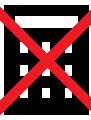a)
(2 marks)

b)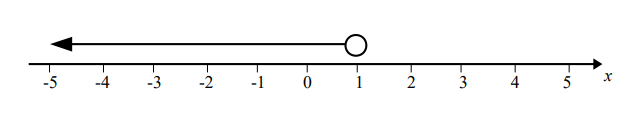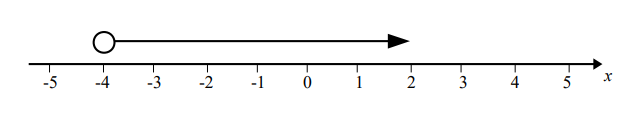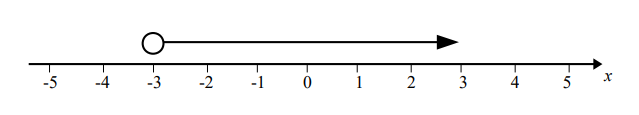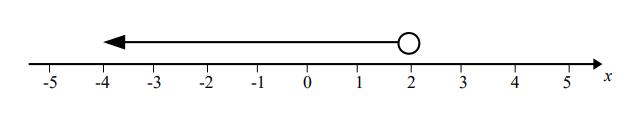(1 mark)

Q2i)

x=
(1 mark)

ii)

x=
(2 marks)

iii)

(2 marks)

Q3Below is a rectangle

i)
(2 marks)

ii)

x= cm
(2 marks)

iii)

cm^2
(2 marks)

Q4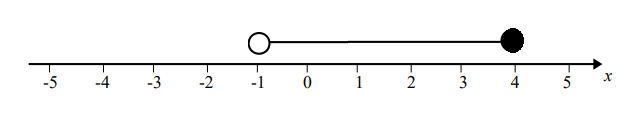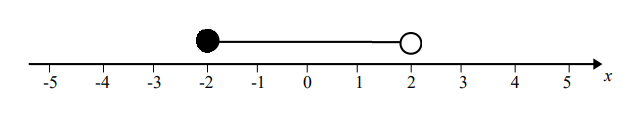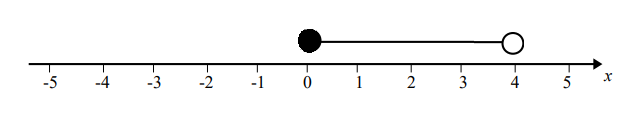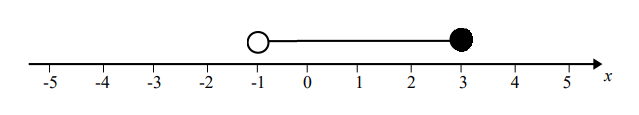(2 marks)

ii)

(3 marks)

Q5p=
(2 marks)

Q6Below is a triangle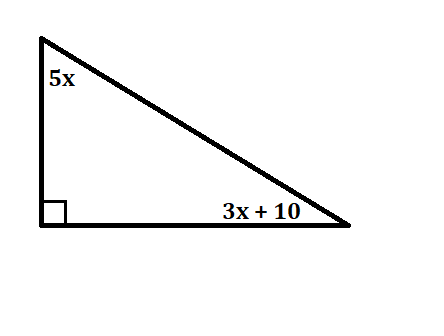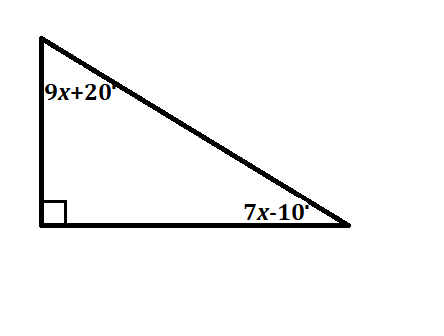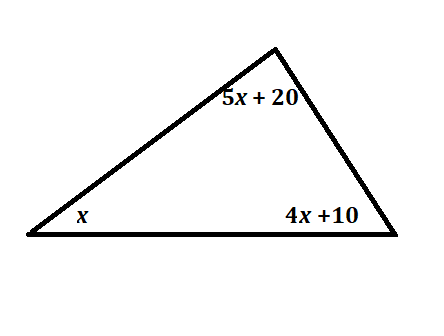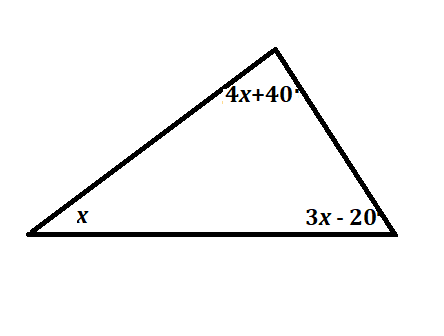i)

x= degrees
(3 marks)

ii)

(1 mark)

Q7(2 marks)

Q8Here is a rectangle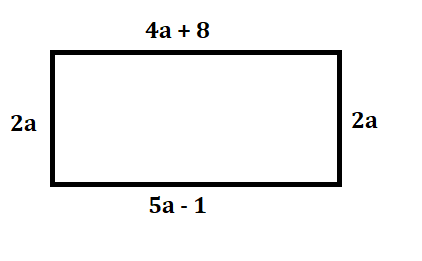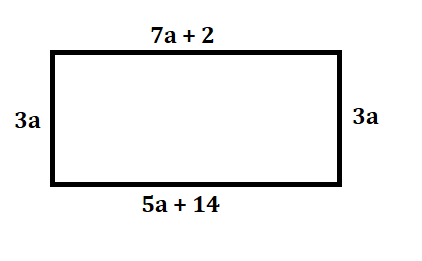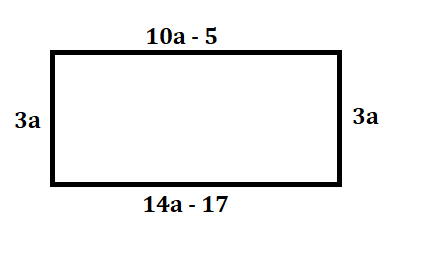i)

cm
(2 marks)

ii)
cm^2
(2 marks)